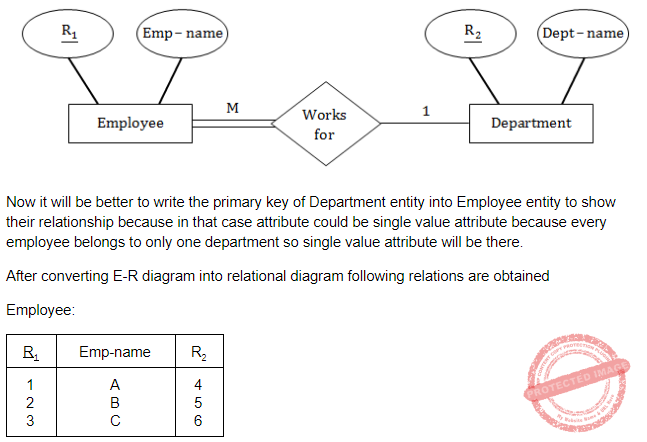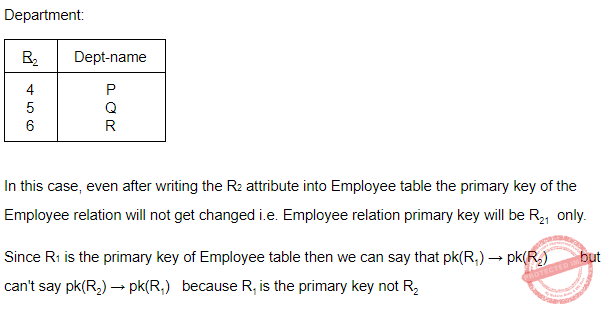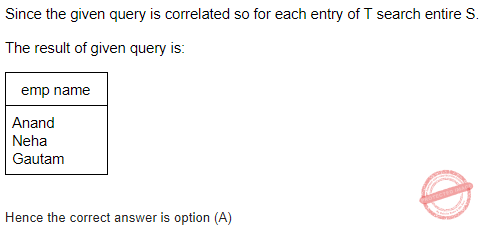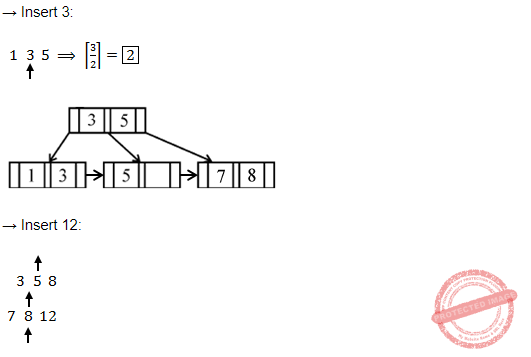## UGC NET CS 2017 Jan- paper-3

 Question 1
Which of the following is an interrupt according to temporal relationship with system clock?
 A Maskable interrupt B Periodic interrupt C Division by zero D Synchronous interrupt
Computer-Organization       Interruption
Question 1 Explanation:
Synchronous interrupts are produced by the CPU control unit while executing instructions and are called synchronous because the control unit issues them only after terminating the execution of an instruction.
This interrupt according to temporal relationship with system clock.
Asynchronous interrupts are generated by other hardware devices at arbitrary times with respect to the CPU clock signals..
Maskable Interrupts - Those interrupts whose request can be denied by microprocessor.
eg- RST 1, RST2, RST 5, RST 6.5 etc..
Non maskable Interrupts - Interrupts whose request cannot be denied. eg -RST 4.5 or TRAP ,RESET IN.
. Periodic interrupts to cause a software task to be executed on a periodic basis.
 Question 2
Which of the following is incorrect for virtual memory?
 A Large programs can be written B More I/O is required C More addressable memory available D Faster and easy swapping of process
Operating-Systems       Virtual Memory
Question 2 Explanation:
Option-A TRUE: Implementing virtual memory is need to write large program
Option-B TRUE: It require more I/O
Option-C FALSE: Not more addressable memory available.
Option-D TRUE: Virtual memory is faster and easy swapping of process.
 Question 3
The general configuration of the micro-programmed control unit is given below:What are blocks B and C in the diagram respectively?
 A Block address register and cache memory B Control address register and control memory C Branch register and cache memory D Control address register and random access memory
Computer-Organization       Microprogrammed-Control-Unit
Question 3 Explanation:Question 4
Match the following :A a-(iv), b-(iii), c-(i), d-(ii) B a-(iv), b-(i), c-(iii), d-(ii) C a-(iv), b-(ii), c-(i), d-(iii) D a-(iv), b-(iii), c-(ii), d-(i)
Question 4 Explanation:
Implied → Specified implicitly in the definition of instruction
Immediate→ Registers which are in CPU
Register→ Specified in the register
Register Indirect→ Specified implicitly in the definition of instruction
 Question 5
In 8085 microprocessor, the digit 5 indicates that the microprocessor needs:
 A –5 volts, +5 volts supply B +5 volts supply only C –5 volts supply only D 5 MHz clock
Computer-Organization       Microprocessor
Question 5 Explanation:
Before 8085 microprocessors require +5V, -5V and 12V. In 8085 microprocessor need +5 volts supply only.
 Question 6
In 8085, which of the following performs : load register pair immediate operation?
 A LDAX rp B LHLD addr C LXI rp, data D INX rp
Computer-Organization       Microprocessor
Question 6 Explanation:
LDAX: Load accumulator indirect( This instruction copies the contents of that memory location into the accumulator. )
LHLD: Load H and L register direct ( This instruction loads the contents of the 16- bit memory location into the H and L register pair. )
LXI: Load register pair immediate( The instruction loads 16-bit data in the register pair designated in the operand.)
INX: Increment register pair by 1.( It will increment the register value by 1.)
 Question 7

Consider following schedules involving two transactions:

S1 : r1(X); r1(Y); r2(X); r2(Y); w2(Y); w1(X)

S2 : r1(X); r2(X); r2(Y); w2(Y); r1(Y); w1(X)

Which of the following statement is true?
 A Both S1 and S2 are conflict serializable. B S1 is conflict serializable and S2 is not conflict serializable. C S1 is not conflict serializable and S2 is conflict serializable. D Both S1 and S2 are not conflict serializable.
Database-Management-System       Transactions
Question 7 Explanation:Question 8
Which one is correct w.r.t. RDBMS ?
 A primary key ⊆ super key ⊆ candidate key B primary key ⊆ candidate key ⊆ super key C super key ⊆ candidate key ⊆ primary key D super key ⊆ primary key ⊆ candidate key
Database-Management-System       Relational-databases
Question 8 Explanation:Question 9
Let pk(R) denotes primary key of relation R. A many-to-one relationship that exists between two relations R1 and R2 can be expressed as follows :
 A pk(R2) → pk(R1) B pk(R1) → pk(R2) C pk(R2) → R1 ∩ R2 D pk(R1) → R1 ∩ R2
Database-Management-System       Keys
Question 9 Explanation:
Consider an example, where we have two entities Employee(whose primary key is R1) and Department(whose primary key is R2) and a department have many employees and each employee works in one department i.e. many to one relationship.Question 10
For a database relation R(A, B, C, D) where the domains of A, B, C and D include only atomic values, only the following functional dependencies and those that can be inferred from them are :
A → C
B → D
The relation R is in _______.
 A First normal form but not in second normal form. B Both in first normal form as well as in second normal form. C Second normal form but not in third normal form. D Both in second normal form as well as in third normal form.
Database-Management-System       Normalization
Question 10 Explanation:
Candidate key is ab.
Since all a, b, c, d are atomic. So the relation is in 1NF.
Checking the FD's
a → c
b → d
We can see that there is partial dependencies. So it is not 2NF.
 Question 11
Works (emp name, company name, salary)
Here, emp_name is primary key. Consider the following SQL query
Select emp name
From works T
Where salary > (select avg (salary)
from works S
where T.company _ name = S.company _name)
The above query is for following :
 A Find the highest paid employee who earns more than the average salary of all employees of his company B Find the highest paid employee who earns more than the average salary of all the employees of all the companies. C Find all employees who earn more than the average salary of all employees of all the companies. D Average salary of all employees of their company.
Database-Management-System       SQL
Question 11 Explanation:Question 12
If following sequence of keys are inserted in a B+ tree with K(=3) pointers: 8, 5, 1, 7, 3, 12, 9, 6 Which of the following shall be correct B+ tree?
 ABCDDatabase-Management-System       B-and-B+-Trees
Question 12 Explanation:Question 13
Which of the following statement(s) is/are correct ?
 A Persistence is the term used to describe the duration of phosphorescence. B The control electrode is used to turn the electron beam on and off. C The electron gun creates a source of electrons which are focused into a narrow beam directed at the face of CRT. D All of the above
Computer-Graphics       Display-System
Question 13 Explanation:
TRUE: Persistence is the term used to describe the duration of phosphorescence. Phosphorescence is a process in which energy absorbed by a substance is released relatively slowly in the form of light.
TRUE: The control electrode is used to turn the electron beam on and off.
TRUE: The electron gun creates a source of electrons which are focused into a narrow beam directed at the face of CRT.
 Question 14
A segment is any object described by GKS commands and data that start with CREATE SEGMENT and Terminates with CLOSE SEGMENT command. What functions can be performed on these segments ?
 A Translation and Rotation B Panning and Zooming C Scaling and Shearing D Translation, Rotation, Panning and Zooming
Computer-Graphics       Transformations
 Question 15
Match the following:A a-i, b-ii, c-iii, d-i B a-i, b-iii, c-ii, d-iv C a-iv, b-iii, c-ii, d-i D a-iv, b-ii, c-i, d-iii
Computer-Graphics       Display-System
 Question 16
Below are the few steps given for scan-converting a circle using Bresenham’s Algorithm. Which of the given steps is not correct ?
 A Compute d = 3 – 2r (where r is radius) B Stop if x > y C If d < 0, then d = 4x + 6 and x = x + 1 D If d ≥, then d = 4 * (x – y) + 10, x = x + 1 and y = y + 1
Computer-Graphics       Bresenham’s-Algorithm
Question 16 Explanation:
Scan converting a circle using Bresenham’s algorithm:
1 Initially X = 0 , Y = R and D = 3 – 2R

2. While (X < Y)

3. Call Draw Circle(Xc, Yc, X, Y)

4. Set X = X + 1

5. If (D < 0) Then

6. D = D + 4X + 6

7. Else

8. Set Y = Y – 1

9. D = D + 4(X – Y) + 10

10. End If

11. Draw Circle(Xc, Yc, X, Y) /* calling function call */

12. End While
 Question 17
Which of the following is/are side effects of scan conversion ?
a. Aliasing
b. Unequal intensity of diagonal lines
c. Overstriking in photographic applications
d. Local or Global aliasing
 A a and b B a, b and c C a, c and d D a, b, c and d
Computer-Graphics       Aliasing
Question 17 Explanation:
Side effects of scan conversions are 1. Aliasing
2. Unequal intensity of diagonal lines
3. Overstriking in photographic applications
4. Local or Global aliasing
 Question 18
Consider a line AB with A=(0,0) and B=(8,4). Apply a simple DDA algorithm and compute the first four plots on this line.
 A [(0, 0), (1, 1), (2, 1), (3, 2)] B [(0, 0), (1, 1.5), (2, 2), (3, 3)] C [(0, 0), (1, 1), (2. 2.5), (3, 3)] D [(0, 0), (1, 2), (2, 2), (3, 2)]
Computer-Graphics       DDA-Algorithm
Question 18 Explanation:There are 18 questions to complete.

Register Now